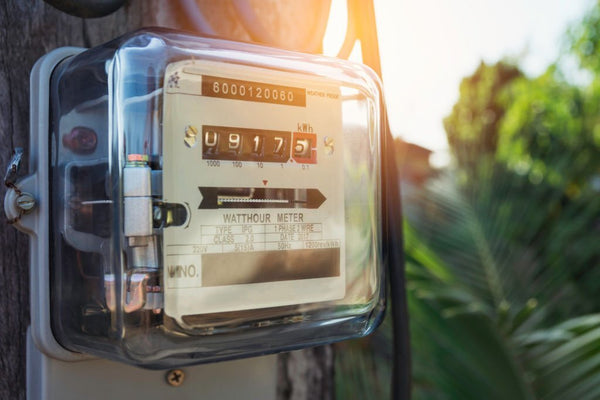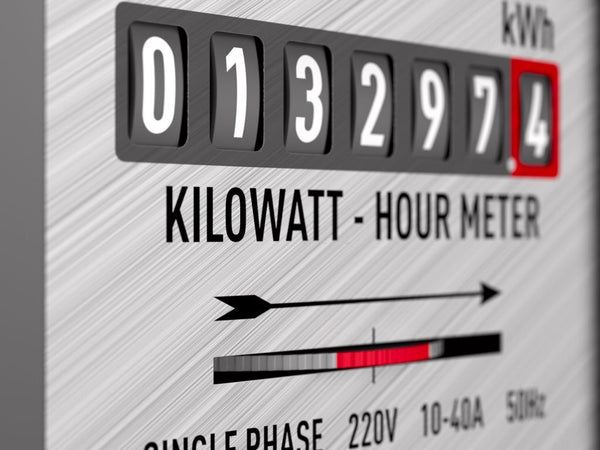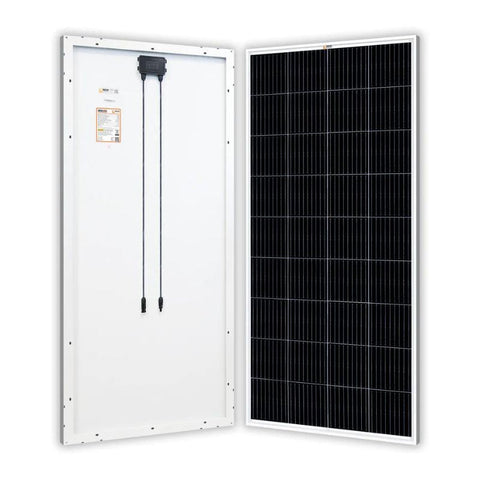Convert Watts to Watt Hours: Understand Your Consumption - ShopSolar.com

# Convert Watts to Watt Hours

When it comes to understanding your electricity needs, you need to be able to convert watts (W) into watt hours (Wh).

Luckily, this is a simple conversion, and it can easily help you better understand your energy consumption. This is especially important if you are looking at investing in a solar-powered system.

Converting watts to watt-hours allows you to understand your energy consumption, and help you plan to meet those needs.

## What Are Watt Hours?

Put simply, a watt-hour is the amount of energy used by 1 watt over the course of 1 hour. The use of watt-hours helps you to understand how much electricity various devices are consuming.While wattage may seem like a random number, it's actually very closely related to the amount of energy that various appliances use. You can use the formula for calculating watt hours to understand exactly how much you are consuming.

This is incredibly useful if you are trying to cut down your energy use. It can also help you to understand how much electricity a solar panel can generate.

### What Is a Watt?

In order to be able to use watt-hours effectively, you need to know that a watt is a unit of energy that describes the rate of flow of energy. Something with a higher wattage will need a higher flow of energy in order to function. There are many different kinds of watt conversions.

As our society becomes more environmentally conscious, we see a growing number of devices that have a lower wattage. A good example of this can be seen in lightbulbs.

LED lightbulbs are up to 90% more efficient than incandescent lightbulbs. In practice, this means that you can replace a 60-watt incandescent bulb with a 10-watt LED one.

## How to Calculate Watt Hours

So how does wattage relate to watt-hours? To calculate watt-hours, you take the wattage of the device and multiply it by the number of hours that it is in use.

This can be written as watt hours = watts x hours, or simplified to Wh = W x h.

A 60-watt incandescent bulb uses 60 watt hours to run for an hour. On the other hand, a more efficient LED bulb would only consume 10-watt-hours.If that same LED light was left on for 6 hours, you would multiply the watts (10) by the number of hours (6), to find out that the lightbulb would consume 60 watt-hours over that time period.

When it comes to finding out the wattage of your devices, most devices will have the wattage written on them or on their box. This is true of household appliances like kettles and toasters.

If you’re trying to better understand heating elements, then you need a watt density calculator, as that deals with the energy requirements for generating heat.

## Why Are Watt Hours Important?

But this is only half of the equation if you’re also using solar energy. When it comes to choosing solar panels and a solar battery, you need to understand how much energy it produces and how long that will last you.

For example, in theory, a 200-watt monocrystalline solar panel produces 200 watts over the course of one hour of direct sunlight. That translates into 200Wh Obviously, you have to take into consideration the efficiency of your panel, and how direct the sunlight is.In practice, a 200-watt solar panel produces around 600Wh of electricity in a day. Because you can work out the Wh usage of your appliances, you can understand if that is enough to meet your needs.

When it comes to using the energy produced by your solar panels, you’ll also need a DC watts to AC watts calculator since the current has to travel through an inverter for everything to work properly.

If you are camping or living in an RV, that might be enough to meet your power needs. It could also be enough if you are supplementing your grid power with solar power.

You might also need to do some other calculations to see how much energy your system produces, like converting watt-hours to milliamp hours or converting watt-hours to amp hours.

Did You Find Our Blog Helpful? Then Consider Checking: